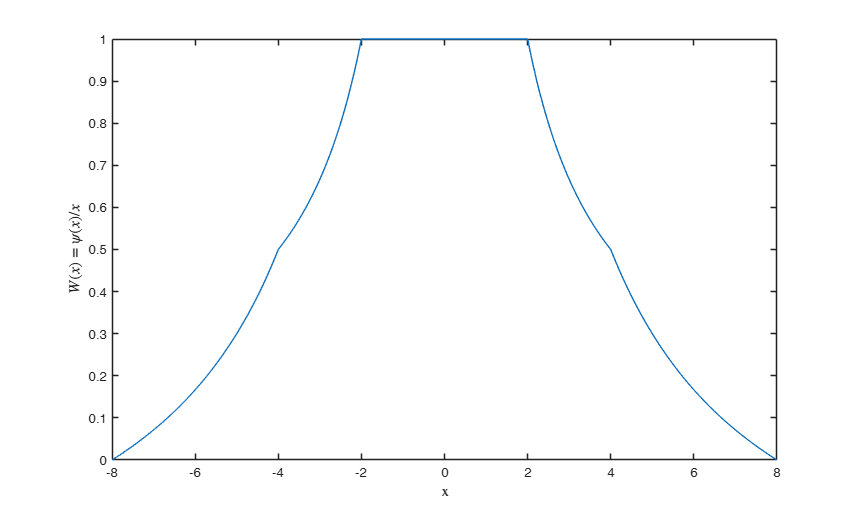# HAwei

HAwei computes weight function psi(u)/u using Hampel proposal

## Syntax

• w=HAwei(u, ctuning)example

## Description

 w =HAwei(u, ctuning) Plot of weight function.

## Examples

expand all

###Plot of weight function.

Obtain Figure 11.15 (panel b) p. 382 of Hoaglin et al. (1987)

x=-8:0.01:8;
weiHA=HAwei(x,[1 2 4 8]);
plot(x,weiHA)
xlabel('x','Interpreter','Latex')
ylabel('$W (x) =\psi(x)/x$','Interpreter','Latex')## Input Arguments

### u — scaled residuals or Mahalanobis distances. Vector.

n x 1 vector containing residuals or Mahalanobis distances for the n units of the sample

Data Types: single| double

### ctuning — tuning parameters. Scalar or Vector.

Scalar or vector of length 4 which specifies the value of the tuning constant c (scalar greater than 0 which controls the robustness/efficiency of the estimator) and the prefixed values of paramters a, b, c:

ctuning(1) = tuning constant which will multiply parameters a, b and c of Hampel rho (psi) function;

ctuning(2) = paramter a of Hampel rho (psi) function;

ctuning(3) = paramter b of Hampel rho (psi) function;

ctuning(4) = paramter c of Hampel rho (psi) function.

Remark: if length(ctuning)==1 values of a, b and c will be set to a=2*ctuning b=4*ctuning c=4*ctuning With these choices, if ctuning=1 the resulting influence function is nearly identical to the biweight with parameter 8.

Data Types: single| double

## Output Arguments

### w —Hampel weights associated to the residuals or Mahalanobis distances for the n units of the sample. n -by- 1 vector

Function HAwei transforms vector u as follows $HAwei(u) = \left\{ \begin{array}{cc} 1 & |u| <= a \\ \frac{a}{|u|} & a \leq |u| < b \\ \frac{a}{|u|} \times \frac{c-|u|}{c-b}, & b <= |u| < c \\ 0 & |u| >= c \end{array} \right.$

where ctun=ctuning(1).

$a$= ctun *ctuning(2).

$b$= ctun *ctuning(3).

$c$= ctun *ctuning(4).

The default is $a$= 2*ctun.

$b$= 4*ctun.

$c$= 8*ctun.

Hoaglin, D.C., Mosteller, F., Tukey, J.W. (1982), "Understanding Robust and Exploratory Data Analysis", Wiley, New York.